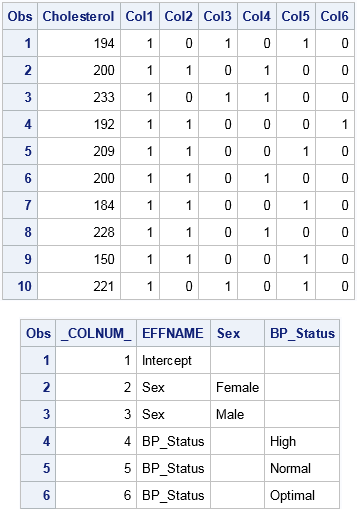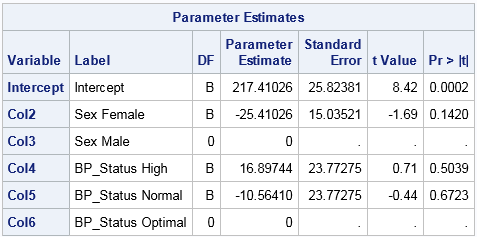A dummy variable (also known as indicator variable) is a numeric variable that indicates the presence or absence of some level of a categorical variable. The word "dummy" does not imply that these variables are not smart. Rather, dummy variables serve as a substitute or a proxy for a categorical variable, just as a "crash-test dummy" is a substitute for a crash victim, or a "sewing dummy" is a dressmaker's proxy for the human body.

In regression and other statistical analyses, a categorical variable can be replaced by dummy variables. For example, a categorical variable with levels "Low," "Moderate," and "High" can be represented by using three binary dummy variables. The first dummy variable has the value 1 for observations that have the level "Low," and 0 for the other observations. The second dummy variable has the value 1 for observations that have the level "Moderate," and zero for the others. The third dummy variable encodes the "High" level.

There are many ways to construct dummy variables in SAS. Some programmers use the DATA step, but there is an easier way. This article discusses the GLMMOD procedure, which produces basic binary dummy variables. A subsequent blog post discusses other SAS procedures that provide alternative methods for representating categorical variables.

### Why generate dummy variables in SAS?

Many programmers never have to generate dummy variables in SAS because most SAS procedures that model categorical variables contain a CLASS statement. If a procedure contains a CLASS statement, then the procedure will automatically create and use dummy variables as part of the analysis.

However, it can be useful to create a SAS data set that explicitly contains a design matrix, which is a numerical matrix that use dummy variables to represent categorical variables. A design matrix also includes columns for continuous variables, the intercept term, and interaction effects. A few reasons to generate a design matrix are:

• Students might need to create a design matrix so that they can fully understand the connections between regression models and matrix computations.
• If a SAS procedure does not support a CLASS statement, you can use often use dummy variables in place of a classification variable. An example is PROC REG, which does not support the CLASS statement, although for most regression analyses you can use PROC GLM or PROC GLMSELECT. Another example is the MCMC procedure, whose documentation includes an example that creates a design matrix for a Bayesian regression model.
• In simulation studies of regression models, it is easy to generate responses by using matrix computations with a numerical design matrix. It is harder to use classification variables directly.

### PROC GLMMOD: Design matrices that use the GLM parameterization

The following DATA step create a data set with 10 observations. It has one continuous variable (Cholesterol) and two categorical variables. One categorical variable (Sex) has two levels and the other (BP_Status) has three levels.

```data Patients; keep Cholesterol Sex BP_Status; set sashelp.heart; if 18 <= _N_ <= 27; run;   proc print; var Cholesterol Sex BP_Status; run;```The GLMMOD procedure can create dummy variables for each categorical variable. If a categorical variable contains k levels, the GLMMOD procedure creates k binary dummy variables. The GLMMOD procedure uses a syntax that is identical to the MODEL statement in PROC GLM, so it is very easy to use to create interaction effects.

The following call to PROC GLMMOD creates an output data set that contains the dummy variables. The output data set is named by using the OUTDESIGN= option. The OUTPARAM= option creates a second data set that associates each dummy variable to a level of a categorical variable:

```proc glmmod data=Patients outdesign=GLMDesign outparm=GLMParm; class sex BP_Status; model Cholesterol = Sex BP_Status; run;   proc print data=GLMDesign; run; proc print data=GLMParm; run;```The OUTDESIGN= data set contains the design matrix, which includes variables named COL1, COL2, COL3, and so forth. The OUTPARM= data set associates levels of the original variables to the dummy variables. For these data, the GLMMOD procedure creates six binary columns. The first is the intercept column. The next two encode the Sex variable. The last three encode the BP_Status variable. If you specify interactions between the original variables, additional dummy variables are created. Notice that the order of the columns is the sort order of the values of their levels. For example, the "Female" column appears before the "Male" column.

When you use this design matrix in a regression analysis, the parameter estimates of main effects estimate the difference in the effects of each level compared to the last level (in alphabetical order). The following statements show that using the dummy variables in PROC REG give the same parameter estimates as are obtained by using the original classification variables in PROC GLM:

```ods graphics off; /* regression analysis by using dummy variables */ proc reg data=GLMDesign; DummyVars: model Cholesterol = COL2-COL6; /* dummy variables except intercept */ ods select ParameterEstimates; quit;   /* same analysis by using the CLASS statement */ proc glm data=Patients; class sex BP_Status; /* generates dummy variables internally */ model Cholesterol = Sex BP_Status / solution; ods select ParameterEstimates; quit;```The parameter estimates from PROC REG is shown. The parameter estimates from PROC GLM are identical. Notice that the parameter estimates for the last level are set to zero and the standard errors are assigned missing values. This occurs because the dummy variable for each categorical variable is redundant. For example, the second dummy variable for the Sex variable ("Males") is a linear combination of the intercept column and the dummy variable for "Females"). Similarly, the last dummy variable for the BP_Status variable ("Optimal") is a linear combination of the intercept column and the "High" and "Normal" dummy variables. By setting the parameter estimate to zero, the last column for each set of dummy variables does not contribute to the model.

For this reason, the GLM encoding is called a singular parameterization. In my next blog post I will present ways to parameterize levels of the categorical variables. These different parameterizations lead to nonsingular design matrices.

The post Create dummy variables in SAS appeared first on The DO Loop.